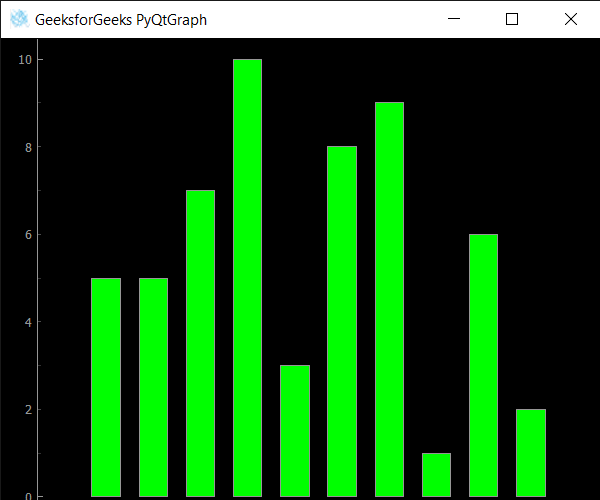# PyQtGraph – Getting Rotation of Bar Graph

• Last Updated : 25 Sep, 2020

In this article we will see how we can get the rotation of the bar graph in the PyQtGraph module. PyQtGraph is a graphics and user interface library for Python that provides functionality commonly required in designing and science applications. Its primary goals are to provide fast, interactive graphics for displaying data (plots, video, etc.) and second is to provide tools to aid in rapid application development (for example, property trees such as used in Qt Designer).A bar chart or bar graph is a chart or graph that presents categorical data with rectangular bars with heights or lengths proportional to the values that they represent. The bars can be plotted vertically or horizontally. A vertical bar chart is sometimes called a column chart. A rotation is a circular movement of an object around a center of rotation. It can be rotated with the help of `rotate` method.

We can create a plot window and bar graph with the help of commands given below

```# creating a pyqtgraph plot window
window = pg.plot()

# creating a bar graph of green color
bargraph = pg.BarGraphItem(x=x, height=y1, width=0.6, brush='g')
```

In order to do this we use `rotation` method with the bar graph object

Syntax : bargraph.rotation()

Argument : It takes no argument

Return : It returns float value

Below is the implementation

 `# importing QtGui to use QIcon ` `from` `PyQt5.QtGui ``import` `*` `from` `PyQt5.QtCore ``import` `Qt  ` ` `  `# importing pyqtgraph as pg ` `import` `pyqtgraph as pg ` ` `  `# importing QtCore and QtGui from the pyqtgraph module ` `from` `pyqtgraph.Qt ``import` `QtCore, QtGui ` ` `  `# importing numpy as np ` `import` `numpy as np ` ` `  `import` `time ` ` `  `# creating a pyqtgraph plot window ` `window ``=` `pg.plot() ` ` `  `# icon for plot window ` `icon ``=` `QIcon(``"logo.png"``) ` ` `  `# setting icon to the plot window ` `window.setWindowIcon(icon) ` ` `  `# setting window geometry ` `# left = 100, top = 100 ` `# width = 600, height = 500 ` `window.setGeometry(``100``, ``100``, ``600``, ``500``) ` ` `  `# title for the plot window ` `title ``=` `"GeeksforGeeks PyQtGraph"` ` `  `# setting window title to plot window ` `window.setWindowTitle(title) ` ` `  `# create list for y-axis ` `y1 ``=` `[``5``, ``5``, ``7``, ``10``, ``3``, ``8``, ``9``, ``1``, ``6``, ``2``] ` ` `  `# create horizontal list i.e x-axis ` `x ``=` `[``1``, ``2``, ``3``, ``4``, ``5``, ``6``, ``7``, ``8``, ``9``, ``10``] ` ` `  `# create pyqt5graph bar graph item  ` `# with width = 0.6 ` `# with bar colors = green ` `bargraph ``=` `pg.BarGraphItem(x ``=` `x, height ``=` `y1, width ``=` `0.6``, brush ``=``'g'``) ` ` `  `# add item to plot window ` `# adding bargraph item to the window ` `window.addItem(bargraph) ` `   `  ` `  `# getting rotation value of the bar graph ` `value ``=` `bargraph.rotation() ` ` `  `# printing the value ` `print``(``"Rotation  : "` `+` `str``(value)) ` ` `  ` `  `# main method ` `if` `__name__ ``=``=` `'__main__'``: ` `     `  `    ``# importing system ` `    ``import` `sys ` `     `  `    ``# Start Qt event loop unless running in interactive mode or using  ` `    ``if` `(sys.flags.interactive !``=` `1``) ``or` `not` `hasattr``(QtCore, ``'PYQT_VERSION'``): ` `        ``QtGui.QApplication.instance().exec_() ` `        `

Output :```Rotation  : 0.0
```

My Personal Notes arrow_drop_up
Recommended Articles
Page :